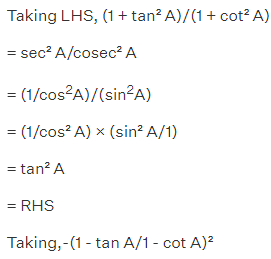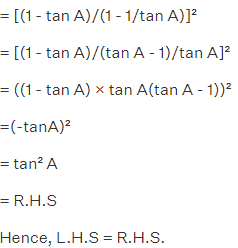FundooTutor

# EXERCISE 8.4

## (vi) √1 + sin A/1 – sin A = sec A+ tan A

LHS = 1 + sin A/(1 – sin A) …..(1)

Multiplying and dividing by (1 + sin A)

⇒ (1 + sin A)(1 + sin A/1 – sin A)(1 + sin A)

= (1 + sin A)²/(1 – sin² A) [a² – b² = (a – b)(a + b)]

= (1 + sinA)/1 – sin² A

= 1 + sin A/cos² A

= 1 + sin A/cos A

= 1/cos A + sin A/cos A

= sec A + tan A

= R.H.S

(vii) (sin θ – 2sin3θ)/(2cos3θ-cos θ) = tan θ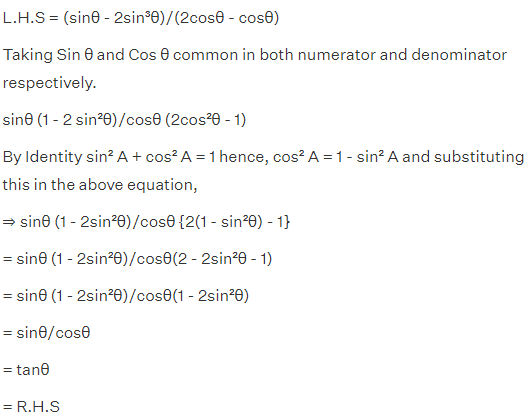(viii) (sin A + cosec A)2 + (cos A + sec A)2 = 7+tan2A+cot2A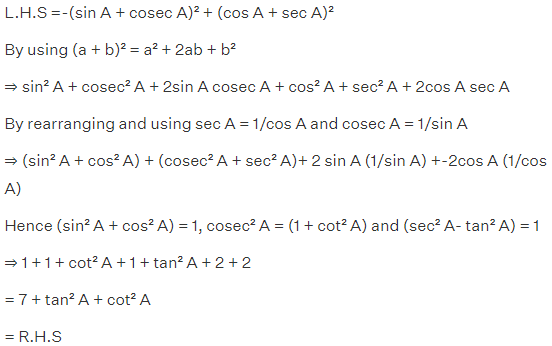(ix) (cosec A – sin A)(sec A – cos A) = 1/(tan A+cotA)

[Hint : Simplify LHS and RHS separately]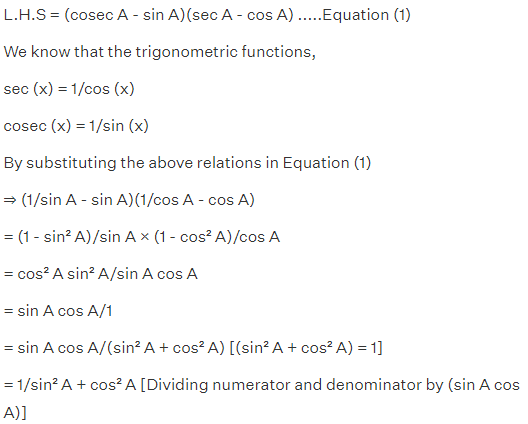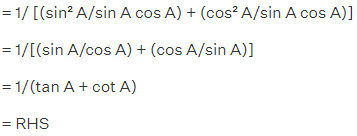(x) (1+tan2A/1+cot2A) = (1-tan A/1-cot A)2 = tan2A